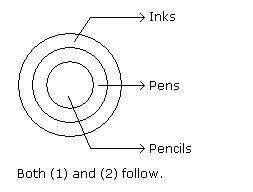Courses

# Test: Syllogism- 1

## 10 Questions MCQ Test UPSC CSAT: Logical Reasoning (LR) & Data Interpretation (DI) | Test: Syllogism- 1

Description
This mock test of Test: Syllogism- 1 for Banking Exams helps you for every Banking Exams entrance exam. This contains 10 Multiple Choice Questions for Banking Exams Test: Syllogism- 1 (mcq) to study with solutions a complete question bank. The solved questions answers in this Test: Syllogism- 1 quiz give you a good mix of easy questions and tough questions. Banking Exams students definitely take this Test: Syllogism- 1 exercise for a better result in the exam. You can find other Test: Syllogism- 1 extra questions, long questions & short questions for Banking Exams on EduRev as well by searching above.
QUESTION: 1

### Instructions: In each of the following questions two statements are given and these statements are followed by two conclusions numbered (1) and (2). You have to take the given two statements to be true even if they seem to be at variance from commonly known facts. Read the conclusions and then decide which of the given conclusions logically follows from the two given statements, disregarding commonly known facts. Q. Statements: Some actors are singers. All the singers are dancers. Conclusions: 1. Some actors are dancers. 2. No singer is actor.

Solution: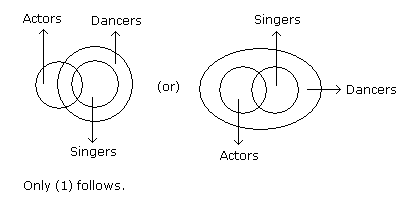QUESTION: 2

### Instructions: In each of the following questions two statements are given and these statements are followed by two conclusions numbered (1) and (2). You have to take the given two statements to be true even if they seem to be at variance from commonly known facts. Read the conclusions and then decide which of the given conclusions logically follows from the two given statements, disregarding commonly known facts. Q. Statements: All the harmoniums are instruments. All the instruments are flutes. Conclusions: 1) All the flutes are instruments. 2) All the harmoniums are flutes.

Solution:

Since all the harmoniums are instruments and all instruments are flutes, then flutes include instruments and harmoniums. And instruments include harmoniums.

(i) All flutes cannot be instruments, because instruments are flutes, but flutes can contain something else as well.

(ii) All the harmoniums are flutes because harmoniums are instruments and instruments are flutes.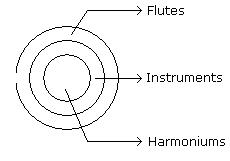QUESTION: 3

### Instructions: In each of the following questions two statements are given and these statements are followed by two conclusions numbered (1) and (2). You have to take the given two statements to be true even if they seem to be at variance from commonly known facts. Read the conclusions and then decide which of the given conclusions logically follows from the two given statements, disregarding commonly known facts. Q. Statements: Some mangoes are yellow. Some tixo are mangoes. Conclusions: 1) Some mangoes are green. 2) Tixo is a yellow.

Solution: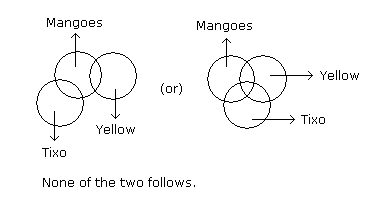QUESTION: 4

InstructionsIn each of the following questions two statements are given and these statements are followed by two conclusions numbered (1) and (2). You have to take the given two statements to be true even if they seem to be at variance from commonly known facts. Read the conclusions and then decide which of the given conclusions logically follows from the two given statements, disregarding commonly known facts.

Q.
Statements:

Some ants are parrots.
All the parrots are apples.

Conclusions:
1. All the apples are parrots.
2.Some ants are apples.

Solution: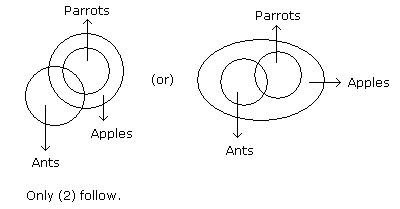QUESTION: 5

InstructionsIn each of the following questions two statements are given and these statements are followed by two conclusions numbered (1) and (2). You have to take the given two statements to be true even if they seem to be at variance from commonly known facts. Read the conclusions and then decide which of the given conclusions logically follows from the two given statements, disregarding commonly known facts.

Q.
Statements:

Some papers are pens.
All the pencils are pens.

Conclusions:
1. Some pens are pencils.
2. Some pens are papers.

Solution:QUESTION: 6

InstructionsIn each of the following questions two statements are given and these statements are followed by two conclusions numbered (1) and (2). You have to take the given two statements to be true even if they seem to be at variance from commonly known facts. Read the conclusions and then decide which of the given conclusions logically follows from the two given statements, disregarding commonly known facts.

Q.
Statements:

All the actors are girls.
All the girls are beautiful.

Conclusions:
1. All the actors are beautiful.
2. Some girls are actors.

Solution: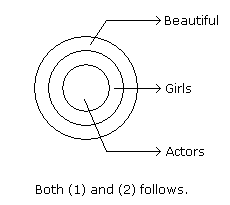QUESTION: 7

InstructionsIn each of the following questions two statements are given and these statements are followed by two conclusions numbered (1) and (2). You have to take the given two statements to be true even if they seem to be at variance from commonly known facts. Read the conclusions and then decide which of the given conclusions logically follows from the two given statements, disregarding commonly known facts.

Q.
Statements:

All the windows are doors.
No door is a wall.

Conclusions:
1. Some windows are walls.
2. No wall is a door.

Solution: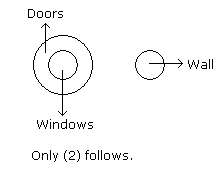QUESTION: 8

InstructionsIn each of the following questions two statements are given and these statements are followed by two conclusions numbered (1) and (2). You have to take the given two statements to be true even if they seem to be at variance from commonly known facts. Read the conclusions and then decide which of the given conclusions logically follows from the two given statements, disregarding commonly known facts.

Q.
Statements:

All cups are books.
All books are shirts.

Conclusions:
1. Some cups are not shirts.
2. Some shirts are cups.

Solution: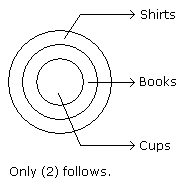QUESTION: 9

InstructionsIn each of the following questions two statements are given and these statements are followed by two conclusions numbered (1) and (2). You have to take the given two statements to be true even if they seem to be at variance from commonly known facts. Read the conclusions and then decide which of the given conclusions logically follows from the two given statements, disregarding commonly known facts.

Q.
Statements:

Some cows are crows.
Some crows are elephants.

Conclusions:
1. Some cows are elephants.
2. All crows are elephants.

Solution: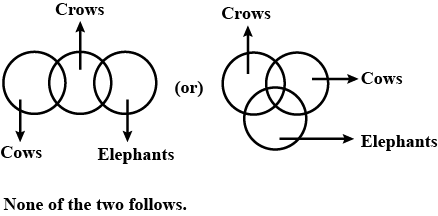QUESTION: 10

InstructionsIn each of the following questions two statements are given and these statements are followed by two conclusions numbered (1) and (2). You have to take the given two statements to be true even if they seem to be at variance from commonly known facts. Read the conclusions and then decide which of the given conclusions logically follows from the two given statements, disregarding commonly known facts.

Q.
Statements:
All the pencils are pens.
All the pens are inks.

Conclusions:
1. All the pencils are inks.
2. Some inks are pencils.

Solution: